# Test: Boolean Algebra & Logic Gates- 2

## 15 Questions MCQ Test GATE Computer Science Engineering(CSE) 2023 Mock Test Series | Test: Boolean Algebra & Logic Gates- 2

Description
Attempt Test: Boolean Algebra & Logic Gates- 2 | 15 questions in 45 minutes | Mock test for Computer Science Engineering (CSE) preparation | Free important questions MCQ to study GATE Computer Science Engineering(CSE) 2023 Mock Test Series for Computer Science Engineering (CSE) Exam | Download free PDF with solutions
QUESTION: 1

### The function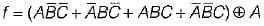can be written as

Solution: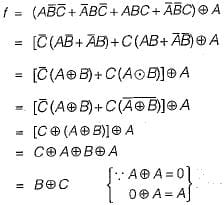QUESTION: 2

### The Karnaugh map for the Boolean function F of 4 Boolean variables is given below where A, B, C are don't care conditions. What values of A, B, C will result in the minimal expression?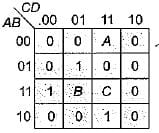Solution:

From the given k-map we can observe that B = 1 always. Also if A is to be paired than it should be
1. If A is selected there is no need to take C.
Hence, A - 1, B = 1, C = 0 Hence option (d) is correct

QUESTION: 3

### Let f(A, B) =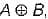then simplified from of the function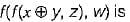Solution: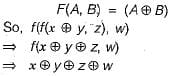QUESTION: 4

Consider the circuit-shown below. Each of the control inputs, C0 through C3, must be tied to a constant, either ‘0’ or '1'.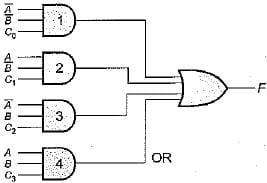What are the values of C0 through C3 that would cause F to be the exclusive OR of A and B?

Solution:

The output of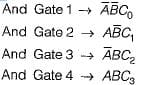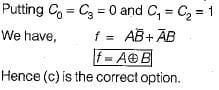QUESTION: 5

Write the minimized expression of the function of three variables that is 1 if the third variable is equal to the OR of the first two variable, 0 otherwise

Solution:

Constructing the table we have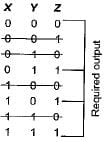Hence the required expression is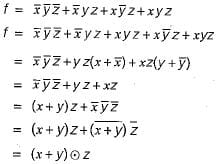QUESTION: 6

Which of the following functions implements the Karnaugh map shown below?Solution:

Solving the given K-map we have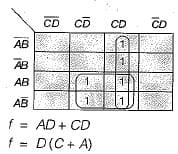Hence (b) is correct option

QUESTION: 7

The Boolean expression for the shaded area in the given Venn diagram is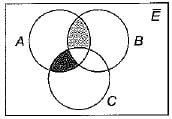Solution:

Hence, the required boolean expression is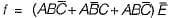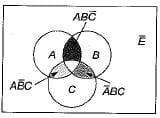Hence (b) is the required option.

QUESTION: 8

The Boolean expression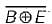is a simplified version of expression: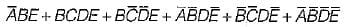Then which of the following choice is correct:
1. Don’t care conditions don’t exist.
2. Don’t care conditions exist.
3. D (16, 18, 20, 23, 27, 29) is the set of don’t care conditions.
​4. D (16, 20, 22, 27, 29) is the set of don’t care conditions.

Solution: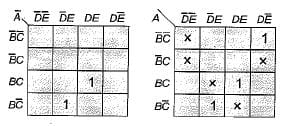Only (c) option satisfies the required condition.

QUESTION: 9

The logical expression for K-Map shown above is: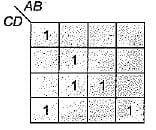Solution: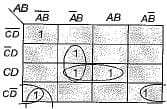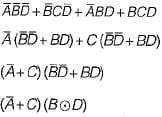QUESTION: 10

The Boolean expression for the shaded area in the Venn diagram is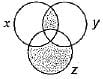Solution: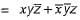QUESTION: 11

Consider, a four variable Boolean function, which contains half the number of minterms with odd number of 1 ’s. Then the Boolean can be realized with variables A, B, C, D as:

Solution:

Constructing the K-map we have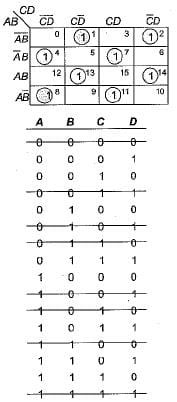Hence the required output is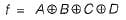QUESTION: 12

Consider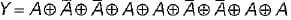the Y is equivalent to:

Solution: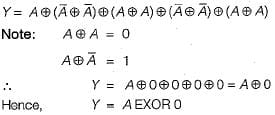QUESTION: 13

An X-Y flip-flop, whose characteristic table is given below, is to be implemented using a JK flip-flop. This can be done by making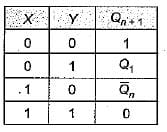Solution: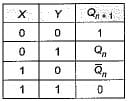The JK fiip-flop can be implemented by making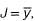, K = x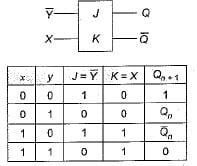Which is true
Hence (d) is correct option.

QUESTION: 14

For what logic gate, the output is complement of the input?

Solution:

By using NOT gate output is complement of input

QUESTION: 15

The NAND can function as a NOT gate if

Solution:

NAND gate work as NOT if inputs are connected together i.e.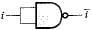Use Code STAYHOME200 and get INR 200 additional OFF Use Coupon Code